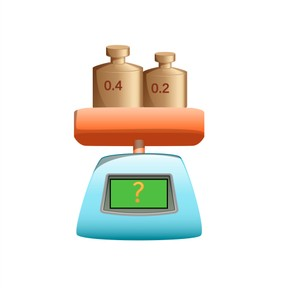Addition with decimal numbers with 1 or 2 decimal places

# Addition with decimal numbers with 1 or 2 decimal places

No account needed.8,000 schools use Gynzy92,000 teachers use Gynzy1,600,000 students use Gynzy

## General

Students learn to add decimal numbers with 1 or 2 decimal places. They also learn to determine which numbers to use from a story problem.

5.NBT.B.7

## Relevance

Discuss with students that it is important to be able to add decimal numbers with 1 or 2 decimal places because you can then calculate how much something costs for example.

## Introduction

Students practice by setting decimal numbers on the number line. Do this by drawing a line from the decimal number to where it belongs on the number line.

## Development

Next comes an exercise in story form. Using the problem with cola bottles, show which steps they should take to solve a story problem. Have them first determine what kind of math problem it is, then determine which numbers are important to the math problem, then to say or write the problem, and finally to solve the problem. Give another story problem and ask students to solve it using the steps given.

Check that students understand adding decimal numbers with 1 or 2 decimal places by asking the following questions:
- Which methods can you use to solve an addition problem with one or two decimal places?
- Which method do you like best? Why?
- How do you solve a story problem?
- Which steps do you take to solve a story problem?

## Guided practice

Students start with an addition problem with a decimal number with one decimal place, then they are given an addition problem with two decimal places, and then they are given a story problem to solve.

## Closing

Check that students are able to say which method they use to solve adding a decimal number with 1 or 2 decimal places. Also have students explain which steps to take to solve a story problem. Check that students have understood by doing a few problems together. Emphasize that students should use the method they feel most comfortable with. Finally they see a number line with a figure at zero. Have the class come up with a number for the figure to jump to. Repeat this again. Then add those two jumps together.
Example: You first select the frog and jump 0.4. You next select the duck and jump 24.5. As a class you solve 0.4+24.5= 24.9

## Teaching tips

Students who have difficulty adding decimal numbers with 1 or 2 decimals can practice with putting decimal numbers on the number line. Give the students a decimal number with 1 decimal point and then ask them to add 0.1 each time and draw that on the number line. Also be sure to practice the jump from 0.9 to 1. Once they are comfortable setting decimal numbers with 1 decimal place they can move to decimal numbers with 2 decimal places.

## Instruction materials

Number line

### The online teaching platform for interactive whiteboards and displays in schools

• Save time building lessons

• Manage the classroom more efficiently

• Increase student engagement